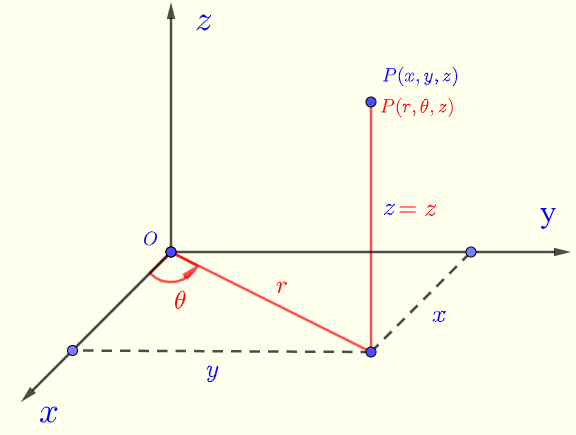# Convert Rectangular to Cylindrical Coordinates - Calculator

## Rectangular and Spherical Coordinates

Convert rectangular to spherical coordinates using a calculator.
It can be shown that the rectangular rectangular coordinates $(x,y,z)$ and cylindrical coordinates $(r,\theta,z)$ in Fig.1 are related as follows:
$x = r \cos \theta$ , $y = r \sin \theta$ , $z = z$       (I)
$r = \sqrt {x^2 + y^2}$ , $\tan \theta = \dfrac{y}{x}$ , $z = z$       (II)
with $0 \le \theta \lt 2\pi$Fig.1 - Rectangular and cylindrical coordinates The calculator calculates the cylindrical coordinates $r$ , $\theta$ and $z$ given the rectangular coordinates $x$ , $y$ and $z$ using the formulas in II.

## Use Calculator to Convert Rectangular to Cylindrical Coordinates

1 - Enter $x$, $y$ and $z$ and press the button "Convert". You may also change the number of decimal places as needed; it has to be a positive integer.
Angle $\theta$ is given in radians and degrees.

 $(x , y , z )$ = (2 , 2 , 3) Number of Decimal Places = 5 $r =$ $\theta =$   Radians $\theta =$   Degrees $z$

## More References and links

Maths Calculators and Solvers.